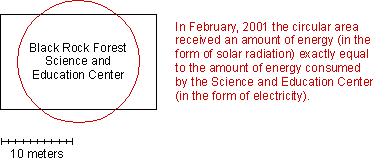### Black Rock Forest Electrical Energy Consumption versus Solar Energy Incidence.

Worksheet #3

This worksheet will guide you in making a quantitative comparison between the electrical energy consumed by the Black Rock Forest Science & Education Center (SEC) and the solar energy received by the Forest.

Directions: Use the pre-selected data tables for February 2001 together with the Conversion Factors and the Fact Sheet. Use Excel or your calculator to find answers.

Step 1. Use the data to find the total amount of electrical energy used by the SEC for February 2001. Explain how you got your answer.

Sum column D of spreadsheet SECelectricity.xls to obtain: ___14,114_____ kWH
(View spreadsheet with answer.)

Step 2: Based on the data, how many kWH of solar energy were incident on each square meter of Black Rock Forest in February 2001? Explain how you got your answer.

Sum Column D of spreadsheet SolarIncidence.xls to obtain: ______62.6_____ kWH/m2
(View spreadsheet with answer.)

Step 3. Use the data to estimate the total amount of solar energy falling on the SEC during Feb 2001. Explain how you got your answer.

Sum column E of spreadsheet SolarIncidence.xls

Or

Multiply answer in Step 2 by area of footprint of SEC

62.6 kWH/m2 * 286 m2 =

____17,900_____ __kWH___ (units)

What are the units of this answer?

Step 4: Based on the data, is the amount of solar energy arriving on the SEC more, less or about the same as the amount of electrical energy used inside the building?

The amount of solar energy (17,900 kHW) incident on the 286m2 footprint of the SEC building in February 2001 was slightly more than the amount of electrical energy consumed by the SEC in the same month (14,114 kWH).

Step 5: In February 2001, how much land area would have been needed to collect sunlight with the same amount of energy as the amount of electrical energy used by the SEC building? Show your calculations.

 Energy used by SEC Incident energy/area = land area needed to collect amount of energy used 14,114 kWH 62.6kWH/m2 = 225 m2

____225______ ___m2____ (units)

What are the units of this answer?

Step 6. Imagine this area as circular in form, and find the radius of the circle with this area.

A = P r 2

r2 = 225 m2 / P = 72 m2

r = 8.5 m

radius = ___8.5 ___ meters

Step 7. On the scaled diagram of the footprint of the SEC, sketch a circle to show the area on which the incident solar energy equals the amount of energy consumed as electricity inside the building.Query: What are your observations? What can you infer from your calculations?

The amount of energy arriving on the building is of the same magnitude as the amount of energy consumed as electricity within the building. Or, conversely, the land area needed to collect energy in the form of sunshine is about the same as the area covered by the building. The implication is that solar energy is a quantitatively plausible source of energy. However, students should be cautioned that energy is lost in the process of converting from one form of energy (solar) to another (electricity), so this calculation gives a maximum amount of energy incident rather than a practical amount of electricity recoverable.

Step 8: Repeat steps 1-6 for the data for the month of June 2001.

Step 9: Finally, we will answer the question, "How much of the electrical power of the building could be supplied by solar panels, if the average solar panel has a 10% efficiency?".

#### Guided Calculation for Solar Panel Efficiency

 1. SI for February (from step 3) __17,900 kWH__ 2. Multiply by footprint of the building x _____286m2_____ = ____5119400____ 3. Multiply by solar efficiency % x .10 = _____511940_____

Could solar panels provide the energy needed by the building?

What percent of the energy needed by the building in February could be provided by solar panels on the roof?

Repeat calculation for the month of June. Answer the same questions.